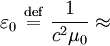## Friday, January 2, 2009

### Could Speed of Light in Intergalactic Space be different?

Here is a thought. Is the speed of light the same in intergalactic space? The measurements of the speed of light seem to always be near stars, either on our end or near the star on the far end. The speed of light is a function of the permittivity and permeability of free space, both of which are derived experimentally and are not calculated from first principles. Could their values turn out to make the speed of light higher in parts of the universe that are not near any matter?

Could the constant speed of light have to do with how it is constant in matter? So the constant speed of light near earth would work for just the same reason speed of light is constant, but at a different speed, in glass and water. Gravity or some other force of nearby matter would be working on a large scale just like it works near atoms on a small scale.

Has anyone actually suggested that as a possibility? If not, why wouldn't that work?

http://en.wikipedia.org/wiki/Free_space

Even in classical physics it was realized  that the vacuum must have a field-dependent permittivity in the strong fields found near point charges.

Vacuum permittivity (also called permittivity of free space or the electric constant) is the ratio D/E in free space.8.8541878176… × 10−12 F/m (or C2N-1m-2),
c (or c0) is the speed of light in free space.

http://en.wikipedia.org/wiki/Permeability_%28electromagnetism%29

Permeability varies with magnetic field. Values shown above are approximate and valid only at the magnetic fields shown. Moreover, they are given for a zero frequency; in practice, the permeability is generally a function of the frequency. When frequency is considered the permeability can be complex, corresponding to the in phase and out of phase response.

http://en.wikipedia.org/wiki/Intergalactic_space#Intergalactic_space

The density of the Universe, however, is clearly not uniform; it ranges from relatively high density in galaxies (including very high density in structures within galaxies, such as planets, stars, and black holes) to conditions in vast voids that have much lower density than the Universe's average. The temperature is only 2.73 Kelvin. NASA's COBE mission(Cosmic Background Explorer) measured the temperature as 2.725 +/- 0.002 K.

Muzza said...

consider a universe with no energy and no matter

in such a universe does the concept of length have many meaning? or the concept of time?

let us introduce a particle

can we now have anything to measure by?

so let us introduce a second particle

then we could say that we can measure something at one particle relative to another

let us double the "distance" between our particles

now let us half that distance

can we say in doing so the particle has gone back in time as the configuration is now indistinguishable from the original two particle arrangement?

if time and distance depend on the existence of things and their arrangement then can we not infer that time and distance concepts depend on the arrangement of the universe?

if intergalactic space has a very low energy gravity denisty then is it not reasonable to think that light might travel at a different speed there than here, where the gravity density is much higher?

if light traveling through intergalactic space is traveling faster than it does through galactic space then woudn't it be reasonable to think that red-shift is not a consequence of expansion but a consequence of light slowing down as it enters a region of higher gravity density?

would this then not be a good explanation for the many inconsitencies we find in expected shift from distant objects including such things as blue shifted light from stars within our own galaxy and blue-red shift pairing in pulsar star binaries?

if the speed of light in intergalactic space is higher than in regions of higher gravity density then doesn't it seem reasonable to think that light travelling through regions of near zero gravity density might approach infinite speed?

and, if so, then don't we have a problem with the very nature of time?

our problem is that we have latched on to "C" with such conviction that nobody will ever challenge the idea that we have it wrong. After all, with an absolutely fixed C we can have an expanding universe and a big bang and a big bang is as good as claiming "God exists" as anything i can think of.